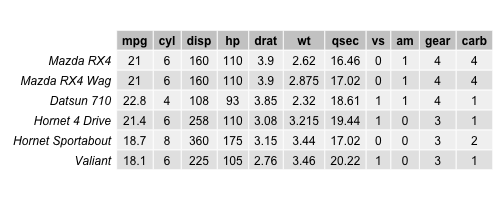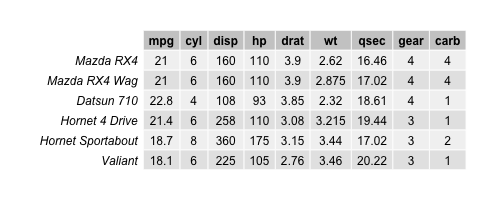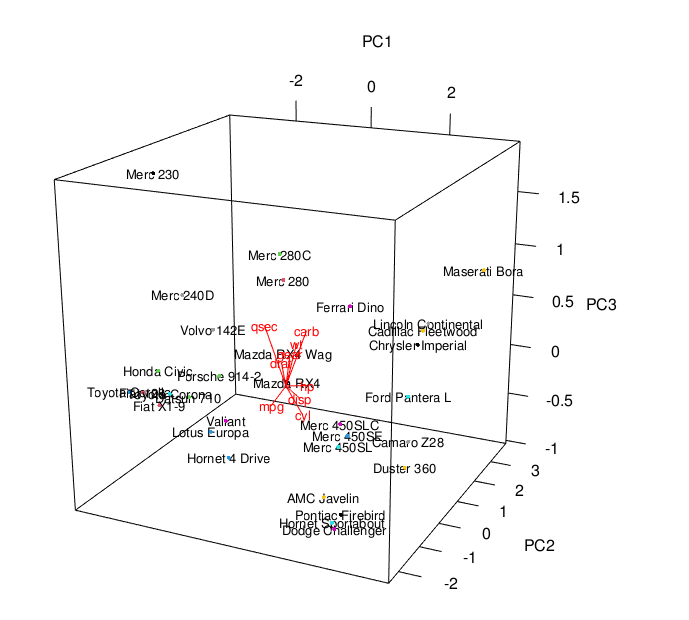# 3D Plot of PCA in R (2 Examples)

In this tutorial, I’ll demonstrate how to draw a 3D Plot of a Principal Component Analysis (PCA) in the R programming language.

This is the table of content:

Here’s how to do it!

First of all, we need to load the library rgl that will allow us to plot our PCA in 3D. If you haven’t installed it, please install it now using the code below.

`install.packages("rgl")`

`library(rgl)`

Now, it’s time to prepare our data in order to perform the PCA. For this example, we will use the mtcars database, which contains 32 car models with 11 features. Let’s take a look at the first rows of the dataset!

```data(mtcars)Even if all variables look numerical, by description, the features `vs` and `am` are categorical by defining if the engine is v-shaped or straight, or if the transmission is manual or automatic. Therefore, those variables are removed from the dataset since PCA essentially works with numeric data. For PCAs deal with categorical data, see our tutorial: Can PCA be Used for Categorical Variables?

```data_cars <- mtcars[,-(8:9),
drop=FALSE]Now, we have a data set with 32 observations and 9 variables. Let’s perform a PCA and see how the data is spread in a 3D plot with respect to the first three principal components!

## Perform PCA

The next step is to perform our PCA in R as follows.

```pca_cars <- prcomp(data_cars,
scale=TRUE)

summary(pca_cars)

# Importance of components:
#                           PC1    PC2     PC3     PC4     PC5     PC6     PC7
# Standard deviation     2.3782 1.4429 0.71008 0.51481 0.42797 0.35184 0.32413
# Proportion of Variance 0.6284 0.2313 0.05602 0.02945 0.02035 0.01375 0.01167
# Cumulative Proportion  0.6284 0.8598 0.91581 0.94525 0.96560 0.97936 0.99103
#                           PC8     PC9
# Standard deviation     0.2419 0.14896
# Proportion of Variance 0.0065 0.00247
# Cumulative Proportion  0.9975 1.00000```

The output shows that the first three principal components explain 92% of the variance. Now, we need to retrieve the component scores too in order to represent the data in a 3D plot on the three principal component axes. The scores are stored under `\$x` in our PCA output. See how we restored them in a data frame named `scores`.

```scores = as.data.frame(pca_cars\$x)Let’s plot the scores in 3D!

## Example 1: Plot PCA in 3D

In order to plot the component scores in 3D, we will use the plot3d() function to plot the observations with respect to the principal component coordinates and the text3d() function to label the car models. Be aware that only the first three columns of the `scores` data set are used, which correspond to the first three components’ scores.

```plot3d(scores[,1:3],
size=5,
col = seq(nrow(scores)))

text3d(scores[,1:3],
texts=c(rownames(scores)),
cex= 0.7, pos=3)```## Example 2: Add Biplot to 3D Plot

We can also add the loading vectors into the previously created 3D scatterplot to obtain a 3D biplot. For this, we will set a for loop that allows us to include the loading vectors showing the relationship between the variables and the three principal components. Please be aware that the loadings are stored in `pca_cars\$rotation`.

```plot3d(scores[,1:3])

text3d(scores[,1:3],
texts=rownames(data_cars),
cex=0.8)

text3d(pca_cars\$rotation[,1:3],
texts=rownames(pca_cars\$rotation),
col="red",
cex=0.8)

coords <- NULL
for (i in 1:nrow(pca_cars\$rotation)) {
coords <- rbind(coords,
rbind(c(0,0,0),
pca_cars\$rotation[i,1:3]))
}

lines3d(coords,
col="red",
lwd=1)```You see the 3D biplot above. If you are also interested in plotting biplots in 2D in R, feel free to visit our tutorial: Biplot of PCA in R.

## Video, Further Resources & Summary

Do you need more explanations and examples for PCA in R? Then you should have a look at the following YouTube video of the Statistics Globe YouTube channel.

Furthermore, you could have a look at some of the other tutorials on Statistics Globe:

This post has shown how to plot your PCA results in 3D. In case you have further questions, you may leave a comment below.

Subscribe to the Statistics Globe Newsletter

•Meseret Muche
July 4, 2023 6:31 pm

remarkable lecture
Q. please share me if you have a video related to NMDS for three or more factors (four sites with varied species)

•July 5, 2023 7:31 am

Hello Meseret,

I am glad that you liked the tutorial. Unfortunately, we don’t have any tutorials on that topic. Let us know if you have any questions regarding PCA.

Best,
Cansu

•Meseret
July 5, 2023 8:01 am

it’s really fascinating and helpful and keep the faith Dr

•July 5, 2023 8:07 am